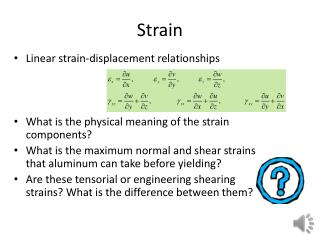DownloadDownload PresentationStrain

# Strain

Télécharger la présentation## Strain

- - - - - - - - - - - - - - - - - - - - - - - - - - - E N D - - - - - - - - - - - - - - - - - - - - - - - - - - -
##### Presentation Transcript

1. Strain • Linear strain-displacement relationships • What is the physical meaning of the strain components? • What is the maximum normal and shear strains that aluminum can take before yielding? • Are these tensorialor engineering shearing strains? What is the difference between them?

2. Stress • Components of the stress tensor • What is the convention with the subscripts of the stress components? • What is a tensor? Give examples of other tensors?

3. Stress-strain relationships • Hooke’s law (Robert Hooke, 1635-1703) an English natural philosopher, architect and polymath. • For most general material • Symmetry from energy considerations (21 coefficients)

4. Isotropic case • Only two independent coefficients • We usually use three: E, G and • Why not two?

5. Isotropic coefficients • Young modulus E(Thomas Young,1773 – 1829) English polymath. Young made notable contributions to the fields of vision, light, solid mechanics, energy, physiology, language, musical harmony, and Egyptology. • Poisson’s ratio Siméon Denis Poisson (1781 – 1840), a Frenchmathematician, geometer, and physicist. • Equations • When do they Blow up?

6. Equilibrium equations • Without body forces • In a cantilever beam under an end load, the normal stress is proportional to the distance from the neutral axis and to the distance from the support. What does it tell you about the shear stress? • We have six stress components and only three equations. How can we solve them?

7. Plane stress • z components of stress are zero . Thin plates • We use plane stress for analyzing stresses in the skins of wings. But they are subject to pressure loads, hence . How come?

8. It is not plane strain! • The strain in the z direction is not zero. We had . So • In upper skin, in-plane stresses tend to be compressive so is tensile, promoting delamination.